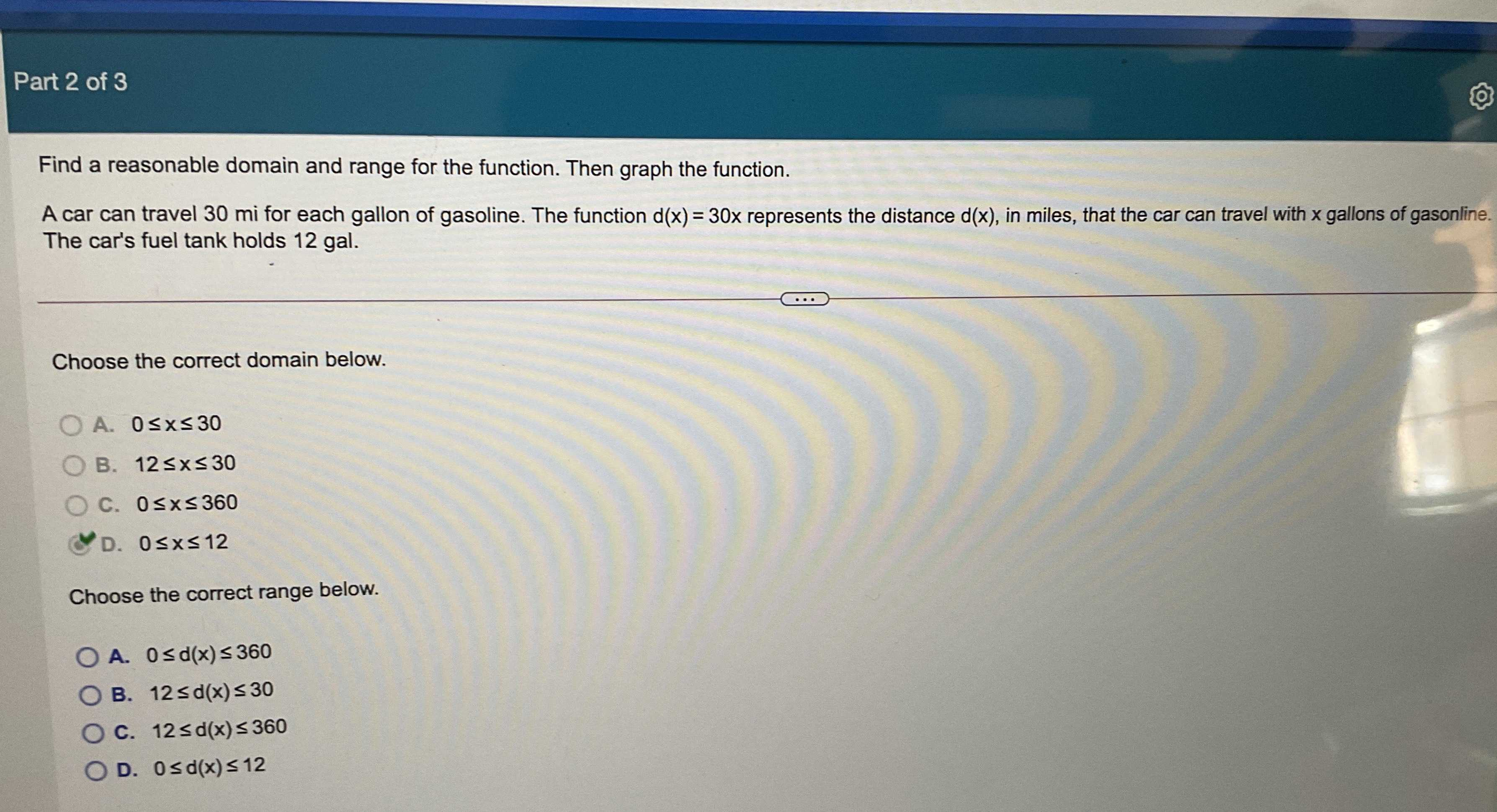### Still have math questions?

Algebra
QuestionFind a reasonable domain and range for the function. Then graph the function. A car can travel $$30$$ mi for each gallon of gasoline. The function $$d ( x ) = 30 x$$ represents the distance $$d ( x )$$ , in miles, that the car can travel with $$x$$ gallons of gasonline. The car's fuel tank holds $$12$$ gal. Choose the correct domain below.

A. $$0 \leq x \leq 30$$

B. $$12 \leq x \leq 30$$

C. $$0 \leq x \leq 360$$

D. $$0 \leq x \leq 12$$

Choose the correct range below.

A. $$0 \leq d ( x ) \leq 360$$

B. $$12 \leq d ( x ) \leq 30$$

C. $$12 \leq d ( x ) \leq 360$$

D. $$0 \leq d ( x ) \leq 12$$